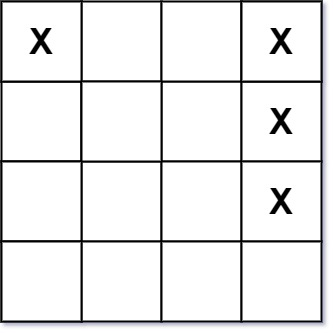## Algorithm

Problem Name: 419. Battleships in a Board

Given an `m x n` matrix `board` where each cell is a battleship `'X'` or empty `'.'`, return the number of the battleships on `board`.

Battleships can only be placed horizontally or vertically on `board`. In other words, they can only be made of the shape `1 x k` (`1` row, `k` columns) or `k x 1` (`k` rows, `1` column), where `k` can be of any size. At least one horizontal or vertical cell separates between two battleships (i.e., there are no adjacent battleships).

Example 1:```Input: board = [["X",".",".","X"],[".",".",".","X"],[".",".",".","X"]]
Output: 2
```

Example 2:

```Input: board = [["."]]
Output: 0
```

Constraints:

• `m == board.length`
• `n == board[i].length`
• `1 <= m, n <= 200`
• `board[i][j]` is either `'.'` or `'X'`.

## Code Examples

### #1 Code Example with Java Programming

```Code - Java Programming```

``````
class Solution {

public int countBattleships(char[][] board) {
int count = 0;
int rows = board.length;
int cols = board.length;
for (int i = 0; i < rows; i++) {
for (int j = 0; j < cols; j++) {
if (board[i][j] == '.' || (i > 0 && board[i - 1][j] == 'X') || (j > 0 && board[i][j - 1] == 'X')) {
continue;
}
count++;
}
}
return count;
}
}
``````
Copy The Code &

Input

cmd
board = [["X",".",".","X"],[".",".",".","X"],[".",".",".","X"]]

Output

cmd
2

### #2 Code Example with Python Programming

```Code - Python Programming```

``````
class Solution:
def countBattleships(self, board):
return sum(board[i][j] == "X" and (i == 0 or board[i - 1][j] == ".") and (j == 0 or board[i][j - 1] == ".") for i in range(len(board)) for j in range(len(board)))
``````
Copy The Code &

Input

cmd
board = [["X",".",".","X"],[".",".",".","X"],[".",".",".","X"]]

Output

cmd
2

### #3 Code Example with C# Programming

```Code - C# Programming```

``````
namespace LeetCode
{
public class _0419_BattleshipsInABoard
{
public int CountBattleships(char[][] board)
{
int rows = board.Length, cols = board.Length;
var count = 0;

for (int i = 0; i < rows; i++)
{
for (int j = 0; j < cols; j++)
{
if (board[i][j] == 'X')
{
if ((i == 0 || board[i - 1][j] != 'X') &&
(j == 0 || board[i][j - 1] != 'X'))
count++;
}
}
}

return count;
}
}
}
``````
Copy The Code &

Input

cmd
board = [["."]]